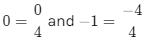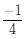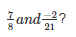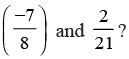Courses

# Test: Rational Numbers

## 10 Questions MCQ Test Class 8 Mathematics by VP Classes | Test: Rational Numbers

Description
This mock test of Test: Rational Numbers for Class 8 helps you for every Class 8 entrance exam. This contains 10 Multiple Choice Questions for Class 8 Test: Rational Numbers (mcq) to study with solutions a complete question bank. The solved questions answers in this Test: Rational Numbers quiz give you a good mix of easy questions and tough questions. Class 8 students definitely take this Test: Rational Numbers exercise for a better result in the exam. You can find other Test: Rational Numbers extra questions, long questions & short questions for Class 8 on EduRev as well by searching above.
QUESTION: 1

Solution:
QUESTION: 2

Solution:
QUESTION: 3

### Which of the following is the identity element of addition?

Solution:

An identity element or neutral element is a special type of element of a set with respect to a binary operation on that set, which leaves any element of the set unchanged when combined with it

An identity with respect to addition is called an additive identity (often denoted as 0) .

QUESTION: 4

Which of the following is the multiplicative identity for rational numbers?

Solution:
QUESTION: 5

Which of the following is neither positive nor a negative rational number?

Solution:
QUESTION: 6

Which of the following rational numbers lies between 0 and –1?

Solution:

Clearly, 0 and −1 cannot lie between 0 and −1.
Also,We can clearly see thatlies between 0 and −1.

QUESTION: 7

Which of the following is the reciprocal of ‘p’?

Solution:
QUESTION: 8

which of the following is the product of?

Solution:
QUESTION: 9

Which of the following is the product ofSolution:
QUESTION: 10

Which of the following is the reciprocal of the reciprocal of a rational number?

Solution: